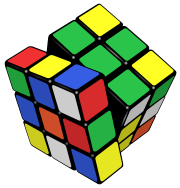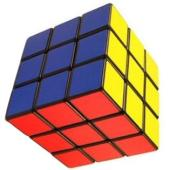A commercially made puzzle, invented by Hungarian Erno Rubik, consisting of a cube of independently rotating faces.

In the starting position each face has 9 stickers of the same colour.

The challenge is to return the cube from a random position to the starting position.There are 43,252,003,274,489,856,000 possible positions of the cube.

The current best algorithm for solution of the cube requires at most 22 moves, and it can be proven by counting arguments that there exist positions needing at least 18 moves to solve.

Such algorithms require great computer resources to execute whereas algorithms used by humans are much longer but far easier to learn and use.

 Video tutorial http://uk.youtube.com/watch?v=LIKEvDRuLAg Printable algorithm http://peter.stillhq.com/jasmine/rubikscubesolution.html Animated cube http://vanderblonk.com/cube/cubeapplet.asp?type=Generator&alg

You can use the animated cube to test the algorithm.

There are championships for solving the Rubik's cube in the shortest time, using one hand and blindfold.

By thinking of each position as a permutation from the starting position, we can think of the set of positions as a group. This group is generated by the collection of "primitive moves".

This is a good example of how a single collection can be thought of in different ways. The actual position is equivalent to the sequence of moves required to get to it from the initial position. That means that a position is equivalent to a permutation, or to a sequence of permutations, etc.

The Rubik's Cube is therefore a good place to explore some fundamental group theory.

As a quick start, the theory of commutators (an element of the form $aba^{-1}b^{-1}$ is a commutator) gives an insight into constructing sequences that move a very small number of the pieces. From that, entire solution algorithms can be devised.